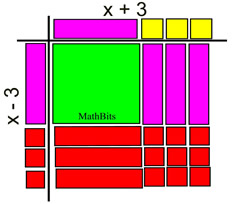Factoring Difference of Two Squares MathBitsNotebook.com Terms of Use   Contact Person: Donna RobertsThere are some special situations that have special methods of factoring. Once such situation is the factoring the difference of two perfect squares. You need to know this formula!Factoring the Difference of Two Squares

We already know that by multiplying:    (a + b)(a - b) = a2 - b2.

If we use the commutative property on this equation we get:

 a2 - b2 = (a + b)(a - b)

which shows a formula for factoring a2 - b2, the difference (subtraction) of two perfect squares.
Notice that the factors are identical except that one is addition and the other is subtraction.In algebra, a term is a perfect square when the numerical coefficient (the number in front of the variable) is a perfect square number, and the exponents of each of the variables are even numbers. 49x2;   25x6;   81y4;   9x2y8;   121x12  are perfect squares.This process of factoring does NOT apply to a2 + b2.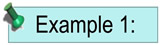Factor: x2 - 64 Solution: Both x2 and 64 are perfect squares and this problem is subtraction. It qualifies for use of the difference of squares formula.   a2 - b2 = (a + b)(a - b) x2 is the square of x; 64 is the square of 8.    a = x and b = 8 [While -x and -8 are also possible, the positive values will make the work easier.] Answer:   x2 - 64 = (x + 8)(x - 8) or (x - 8)(x + 8)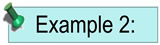Factor: 9m2 - 81n6 Solution: First, there is a common factor of 9 in these terms. 9(m2 - 9n6 ) Both m2 and 9n6 are perfect squares and this problem is subtraction. It qualifies for use of the difference of squares formula.   a2 - b2 = (a + b)(a - b) m2 is the square of m; 9n6 is the square of 3n3.    a = m and b = 3n3 Answer:   9m2 - 81n6 = 9(m + 3n3 )(m - 3n3 )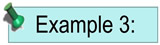Factor: x2 - 9 using Algebra Tiles
KEY: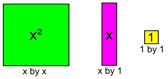See more about Algebra Tiles.
 Place the x2 tile and the -9 (red) tiles in the grid.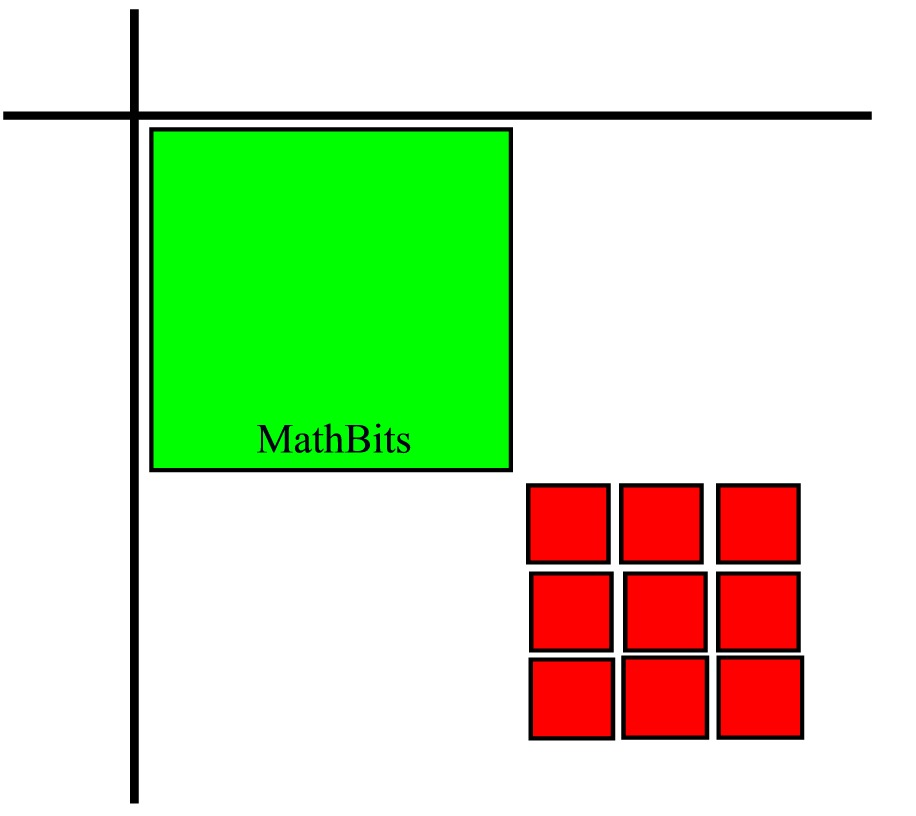Fill in the empty spaces with x-tiles that do not change the value of the current entries. (1 x-tile + 1 red x-tile = 0).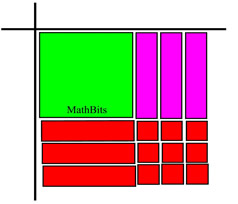Fill the outside sections of the grid with x-tiles and 1-tiles that complete the pattern.